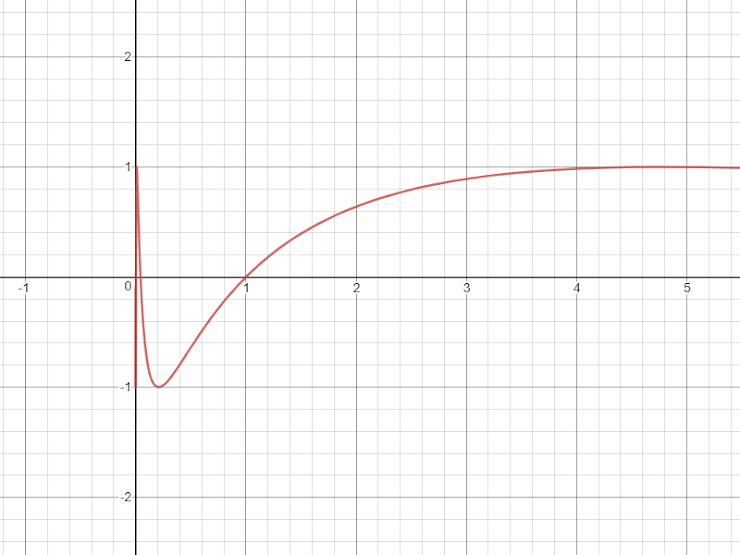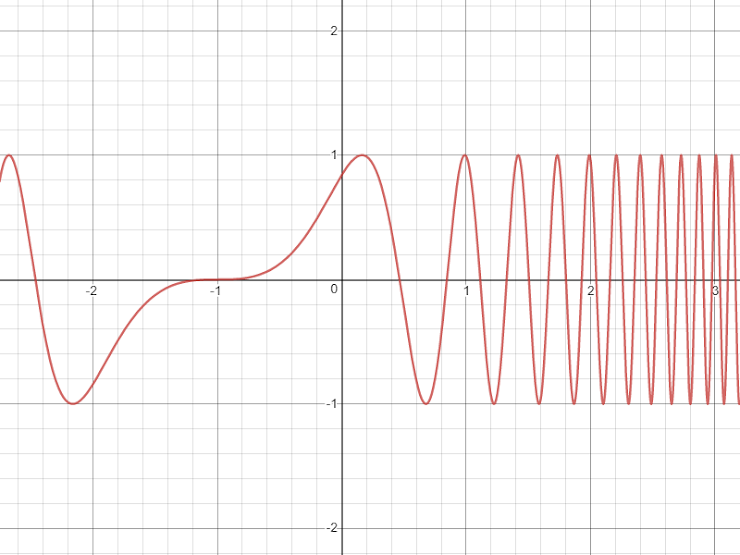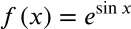Exit Ticket for Day 28 of Calculus
Please select your name from the following list: *
1. Consider the following graph. At x=1, the FIRST DERIVATIVE of this function is apparently ______.2. Using the same graph as in the previous question, at x=1 the SECOND DERIVATIVE of the function is apparently _____.
3. Using the same graph as in the previous question, there is a local minimum of the function at x=0.208. Looking at the graph, the SECOND DERIVATIVE of the function at x=0.208 is apparently _________.
4. On the following graph, where do you see an "inflection point"?These are review questions:
5. What is the derivative of this function? NOTE: All of the options below have a mistake-- the left side of each of them should be f'(x) not f(x).This form was created inside of Evansville Vanderburgh School Corporation. Report Abuse - Terms of Service